Next: Worked Example 4: AC Up: Magnetic Induction Previous: Worked Example 2: Lenz's

## Worked Example 3: Motional emf

Question: Consider the circuit described in Sect. 9.4. Suppose that the length of the moving rod is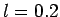m, its speed is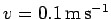, the magnetic field-strength is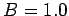T (the field is directed into the page--see Fig. 36), and the resistance of the circuit is. What is the emf generated around the circuit? What current flows around the circuit? What is the magnitude and direction of the force acting on the moving rod due to the fact that a current is flowing along it? What is the rate at which work must be performed on the rod in order to keep it moving at constant velocity against this force? What is the rate at which electrical energy is generated? What is the rate at which energy is converted into heat due to the resistivity of the circuit?

Answer: The emf is generated by the motion of the rod. According to Eq. (204), the magnitude of the motional emf is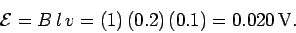The emf acts in the anti-clockwise direction in Fig. 36.

The anti-clockwise current driven around the circuit by the motional emf follows from Ohm's law: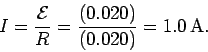Since the rod carries a currentwhich flows perpendicular to a magnetic field, the force per unit length acting on the rod is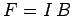(see Sect. 8.2). Thus, the total force acting on the rod is of magnitude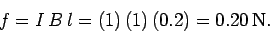This force is directed parallel to the vector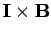. It follows that the force is to the left in Fig. 36. In other words, the force opposes the motion producing the emf.

In order to keep the rod moving at a constant velocity, some external agent must apply a force to the rod which is equal and opposite to the magnetic force described above. Thus, the externally applied force acts to the right. The rate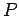at which work is done by this force is the product of the force and the velocity of the rod in the direction of this force. Thus,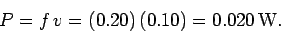Every chargewhich circulates around the circuit in the anti-clockwise direction acquires the energy. The amount of charge per unit time which circulates around the circuit is, by definition, equal to the currentflowing around the circuit. Thus, the rate at which electric charges acquire energy in the circuit is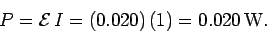Now, the rate at which electric charges acquire energy in the circuit is equal to the rate at which mechanical work is done on the rod by the external force, as must be the case if energy is to be conserved. Thus, we can think of this circuit as constituting a primitive generator which transforms mechanical into electrical energy.

The rate at which electrical energy is converted into heat energy in the circuit is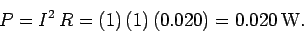Thus, all of the mechanical work done on the rod eventually ends up as heat dissipated in the circuit.Next: Worked Example 4: AC Up: Magnetic Induction Previous: Worked Example 2: Lenz's
Richard Fitzpatrick 2007-07-14